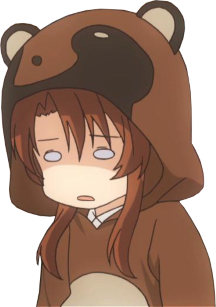# danieljon.es

 index posts opinions portfolio

# Posts

My posts about programming and things.
Date format is day/month/year because I'm sane.

# Bit manipulation

### 3/11/2018

Bit manipulation is something I find interesting, to strengthen my understanding I thought it'd be best to put some of the basics in text.

First, an understanding of binary is required:

128
64 32 16 8 4 2 1
0 0 1 0 1 1 0 0
= 44

Got it? I knew you would. If not see my post on binary.

Next you need an understanding of bitwise operations:
AND (&) ```copies bit if it is set in both operands 11 AND 5 (1011 AND 0101) = 1 1011 0101 ---- 0001 ``` ```inclusive OR, copies bit if it is set in either operand 13 OR 7 (1101 OR 0111) = 15 1101 0111 ---- 1111 ``` ```exclusive OR, copies bit if it is set in either operand but not if it is set in both 13 XOR 7 (1101 XOR 0111) = 10 1101 0111 ---- 1010 ``` ```flips each bit obtaining the numbers one's complement NOT 5 (101) = 2 101 --- 010 ``` ```Shifts the bits of the left operand by the number in the right operand to the left 5 << 2 (101 << 010) = 20 000101 <<< (the 101 is moved to the left by 2 bits) ------ 010100 ``` ```Shifts the bits of the left operand by the number in the right operand to the right, underflow is discarded 22 >> 3 (10110 >> 00011) = 2 10110 >>>>>(the 10110 is move to the right by 3 bits) ----- 00010 ```
Now that those are understood, let's take a look at some bit manipulation, I'm going to use C bitwise operators from now on.

First note, counting bits starts from the least significant bit (right-most), which is the 0th bit.
Setting a bit ```To set a bit to 1 OR the number with 1 left shifted with the desired bit to set number |= (1 << bit) number = 5, bit = 3 5 | (1 << 3) = 13 1 << 3 = 8 0001 <<< ----- 1000 5 | 8 = 13 0101 | 1000 ------ 1101 ^ our 3rd bit (0,1,2,3) is now set ``` ```To clear a bit AND the number with the one's complement of 1 left shifted with the desired bit to clear number &= ~(1 << bit) number = 9, bit = 3 9 & ~(1 << 3) = 1 1 << 3 = 8 0001 <<< ---- 1000 ~8 = 7 1000 ~ ------ 0111 9 & 7 = 1 1001 & 0111 ------ 0001 ^ our 3rd bit (0,1,2,3) is now 0 ``` ```To get a bit right shift the number by the desired bit to retrieve, then AND it by 1 result = (number >> bit) & 1 number = 14, bit = 3 result = (14 >> 3) & 1 result = 1 14 >> 3 = 1 1110 >>> ----- 0001 1 & 1 = 1 01 01 -- 01 ``` ```To toggle a bit XOR the number by 1 left shifted by the desired bit number ^= 1 << bit number = 12, bit = 4 12 ^= 1 << 4 = 28 1 << 4 = 16 00001 <<<< ----- 10000 12 ^ 16 = 28 01100 ^ 10000 ------- 11100 ^ our 4th bit (0,1,2,3,4) is now set ```
Let's do something with this knowledge and use the bits of a char as individual flags that we can use. Using an enum is a much better way to do this.
```#include <stdio.h>

int
dectobin(int dec)
{
/* return the binary representation of dec */
if (dec == 0) return 0;
if (dec == 1) return 1;
return (dec % 2) + 10 * dectobin(dec / 2);
}

int
getbit(int num, int bit)
{
return (num >> bit) & 1;
}

int
togglebit(int number, int bit)
{
return number ^= 1 << bit;
}

int
setbit(int number, int bit)
{
return number |= 1 << bit;
}

int
clearbit(int number, int bit)
{
return number &= ~(1 << bit);
}

int
main(void)
{
unsigned char flags = 0; /* char because it is 1 byte, unsigned because we don't need negatives */
printf("flag has value %d, in binary: %08d\n", flags, dectobin(flags));
puts("let's make the number 145");
puts("we need to toggle bits: 0, 4, 7");
flags = setbit(flags, 0);
puts("set bit 0");
printf("flag has value %d, in binary: %08d\n", flags, dectobin(flags));
flags = setbit(flags, 4);
puts("set bit 4");
printf("flag has value %d, in binary: %08d\n", flags, dectobin(flags));
flags = setbit(flags, 7);
puts("set bit 7");
printf("flag has value %d, in binary: %08d\n", flags, dectobin(flags));

puts("let's check the value of bit 7");
printf("bit 7 is %d\n", getbit(flags, 7));
puts("we don't want bit 7 set anymore");
flags = clearbit(flags, 7);
puts("cleared bit 7");
printf("flag has value %d, in binary: %08d\n", flags, dectobin(flags));
return 0;
}

```
output:
```flag has value 0, in binary: 00000000
let's make the number 145
we need to toggle bits: 0, 4, 7
set bit 0
flag has value 1, in binary: 00000001
set bit 4
flag has value 17, in binary: 00010001
set bit 7
flag has value 145, in binary: 10010001
let's check the value of bit 7
bit 7 is 1
we don't want bit 7 set anymore
cleared bit 7
flag has value 17, in binary: 00010001
```

page generated 20/8/2022 using websitegenerator in C